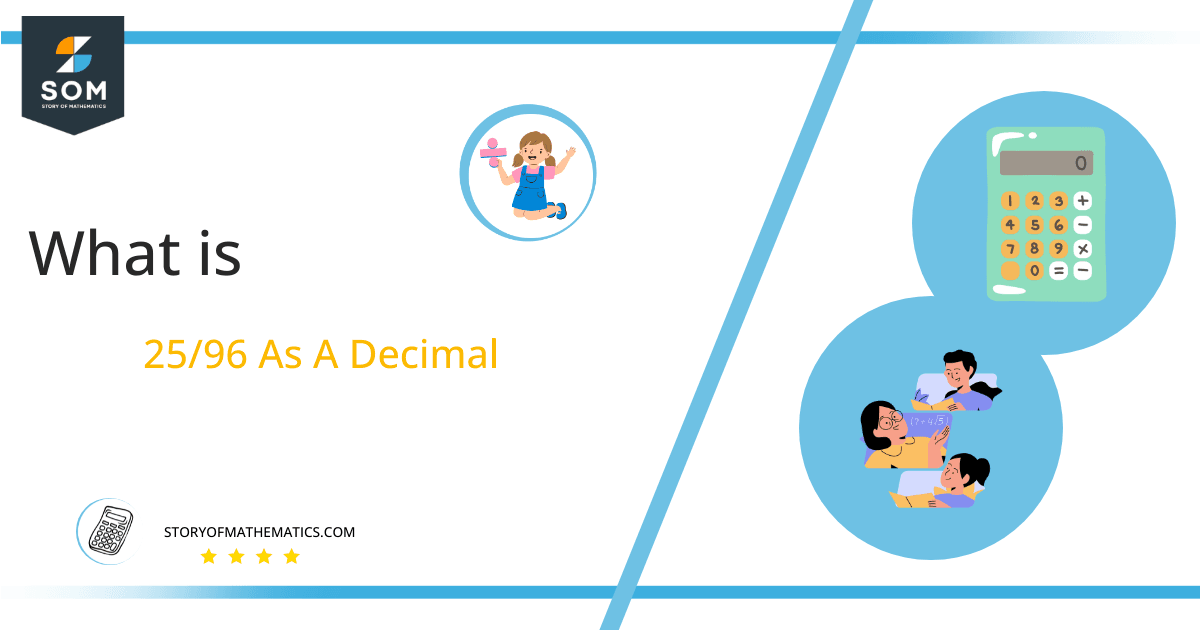# What Is 25/96 as a Decimal + Solution With Free Steps

The fraction 25/96 as a decimal is equal to 0.260.

Rational numbers are numbers that can be expressed in the form of ratios. It is a fraction in which the numerator and denominator are polynomials and represent real numbers. We get Terminating and Recurring decimals when we divide a rational fraction.Here, we are more interested in the division types that result in a Decimal value, as this can be expressed as a Fraction. We see fractions as a way of showing two numbers having the operation of Division between them that result in a value that lies between two Integers.

Now, we introduce the method used to solve said fraction to decimal conversion, called Long Division, which we will discuss in detail moving forward. So, let’s go through the Solution of fraction 25/96.

## Solution

First, we convert the fraction components, i.e., the numerator and the denominator, and transform them into the division constituents, i.e., the Dividend and the Divisor, respectively.

This can be done as follows:

Dividend = 25

Divisor = 96

Now, we introduce the most important quantity in our division process: the Quotient. The value represents the Solution to our division and can be expressed as having the following relationship with the Division constituents:

Quotient = Dividend $\div$ Divisor = 25$\div$ 96

This is when we go through the Long Division solution to our problem.Figure 1

## 25/96 Long Division Method

We start solving a problem using the Long Division Method by first taking apart the division’s components and comparing them. As we have 25 and 96, we can see how 25 is Smaller than 96, and to solve this division, we require that 25 be Bigger than 96.

This is done by multiplying the dividend by 10 and checking whether it is bigger than the divisor or not. If so, we calculate the Multiple of the divisor closest to the dividend and subtract it from the Dividend. This produces the Remainder, which we then use as the dividend later.

Now, we begin solving for our dividend 25, which after getting multiplied by 10 becomes 250.

We take this 250 and divide it by 96; this can be done as follows:

250 $\div$ 96 $\approx$ 2

Where:

96 x 2 = 192

This will lead to the generation of a Remainder equal to 250 – 192 = 58. Now this means we have to repeat the process by Converting the 58 into 580 and solving for that:

580 $\div$ 96 $\approx$ 6

Where:

96 x 6 = 576

This, therefore, produces another Remainder which is equal to 580 – 576 = 4.

Finally, we have a Quotient generated after combining the three pieces of it as 0.260=z, with a Remainder equal to 400.Images/mathematical drawings are created with GeoGebra.